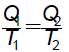Courses

# Second Law of Thermodynamics, Entropy - MCQ Test 2

## 30 Questions MCQ Test RRB JE for Mechanical Engineering | Second Law of Thermodynamics, Entropy - MCQ Test 2

Description
This mock test of Second Law of Thermodynamics, Entropy - MCQ Test 2 for Mechanical Engineering helps you for every Mechanical Engineering entrance exam. This contains 30 Multiple Choice Questions for Mechanical Engineering Second Law of Thermodynamics, Entropy - MCQ Test 2 (mcq) to study with solutions a complete question bank. The solved questions answers in this Second Law of Thermodynamics, Entropy - MCQ Test 2 quiz give you a good mix of easy questions and tough questions. Mechanical Engineering students definitely take this Second Law of Thermodynamics, Entropy - MCQ Test 2 exercise for a better result in the exam. You can find other Second Law of Thermodynamics, Entropy - MCQ Test 2 extra questions, long questions & short questions for Mechanical Engineering on EduRev as well by searching above.
QUESTION: 1

Solution:
QUESTION: 2

Solution:
QUESTION: 3

### Assertion (A): If a graph is plotted for absolute temperature as a function of entropy, the area under the curve would give the amount of heat supplied. Reason (R): Entropy represents the maximum fraction of work obtainable from heat per degree drop in temperature.

Solution:
QUESTION: 4

When a process becomes irreversible due to heat interaction between system and surrounding at the boundary due to finite temperature gradient, then the irreversibility is______

Solution:

All the spontaneous processes are irreversible in nature. The irreversibility is caused by finite potential gradient like temperature gradient etc. or by any dissipative effect like friction. There are two types of irreversibility

i.) Internal irreversibility
ii.) External irreversibility

The external irreversibility occurs due to the temperature difference between the source and a working fluid at heat supply and the temperature difference between the sink and the working fluid at heat rejection. If the hypothetical heat source and sink is considered then the process becomes reversible.

QUESTION: 5

Consider the following statements:

In an irreversible process

1. Entropy always increases.

2. The sum of the entropy of all the bodies taking part in a process always increases.

3. Once created, entropy cannot be destroyed.

Of these statements

Solution:

In irreversible heat rejection process entropy decreases. In an irreversible process entropy of the universe always increases i.e. sum of system + surroundings will increases.

Consider the process 3–4 if it is irreversible process then also entropy will decrease.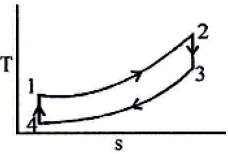QUESTION: 6

A system of 100 kg mass undergoes a process in which its specific entropy increases from 0.3 kJ/kg-K to 0.4 kJ/kg-K. At the same time, the entropy of the surroundings decreases from 80 kJ/K to 75 kJ/K. The process is:

Solution:

Entropy increase in process = 100 (0.4 – 0.3) = 10 kJ/kg
Entropy change of surroundings = 5 kJ/K
Thus net entropy increases and the process is irreversible

QUESTION: 7

The entropy of a mixture of ideal gases is the sum of the entropies of constituents evaluated at:

Solution:
QUESTION: 8

One kg of air is subjected to the following processes:

1. Air expands isothermally from 6 bar to 3 bar.

2. Air is compressed to half the volume at constant pressure

3. Heat is supplied to air at constant volume till the pressure becomes three fold

In which of the above processes, the change in entropy will be positive?

Solution: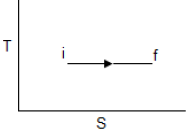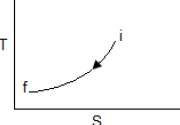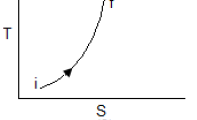QUESTION: 9

In which one of the following situations the entropy change will be negative

Solution:

It is isobaric compression.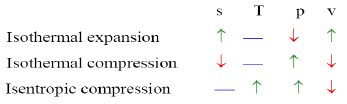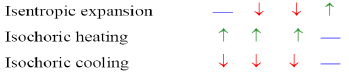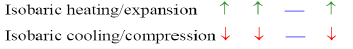QUESTION: 10

Increase in entropy of a system represents

Solution:
QUESTION: 11

Heat flows between two reservoirs having temperatures 1000 K and 500 K, respectively. If the entropy change of the cold reservoir is 10 kJ/K, then what is the entropy change for the hot reservoir?

Solution: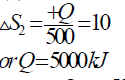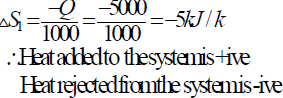QUESTION: 12

Which one of the following pairs best expresses a relationship similar to that expressed in the pair “pressure-volume” for a thermodynamic system undergoing a process?

Solution:

That so why we are using p–v or T–s diagram.

QUESTION: 13

In the T-S diagram shown in the figure, which one of the following is represented by the area under the curve?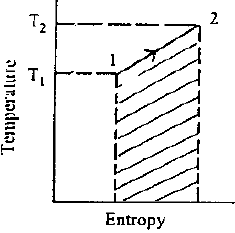Solution:
QUESTION: 14

If a system undergoes an irreversible adiabatic process, then (symbols have usual meanings)

Solution: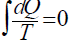does not necessarily means reversible process. If dQ = 0 .

QUESTION: 15

Which one of the following statements is not correct?

Solution:

Throttling is a constant enthalpy expansion process.

QUESTION: 16

1600 kJ of energy is transferred from a heat reservoir at 800 K to another heat reservoir at 400 K. The amount of entropy generated during the process would be:

Solution: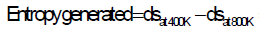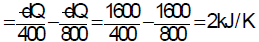QUESTION: 17

M1 kg of water at T1 is isobarically and adiabatically mixed with M2 kg of water at T2 (T1 > T2). The entropy change of the universe is:

Solution:
QUESTION: 18

Nitrogen gas (molecular weight 28) is enclosed in a cylinder by a piston, at the initial condition of 2 bar, 298 K and 1 m3. In a particular process, the gas slowly expands under isothermal condition, until the volume becomes 2m3. Heat exchange occurs with the atmosphere at 298 K during this process.

Q.

The work interaction for the Nitrogen gas is:

Solution: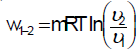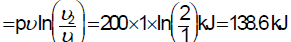QUESTION: 19

If a closed system is undergoing an irreversible process, the entropy of the system

Solution:
QUESTION: 20

In an experimental set-up, air flows between two stations P and Q adiabatically. The direction of flow depends on the pressure and temperature conditions maintained at P and Q. The conditions at station P are 150 kPa and 350 K. The temperature at station Q is 300 K.

The following are the properties and relations pertaining to air:

Specific heat at constant pressure, cp = 1.005 kJ/kg K;

Specific heat at constant volume, cv = 0.718 kJ/kg K;

Characteristic gas constant, R = 0.287 kJ/kg K.

Enthalpy, h = cpT

Internal energy, u = cvT

Q.

If the pressure at station Q is 50 kPa, the change in entropy in kJ/kg  K is

Solution: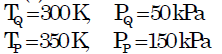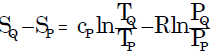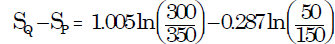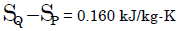QUESTION: 21

Which point is taken as the standard reference point to define the Kelvin temperature scale?

Solution:

The absolute thermodynamic temperature scale is also known as the Kelvin temperature scale. As the heat is absorbed and rejected respectively by the Carnot engine operating between two reservoirs of different temperatures T1 and T2, these two temperatures on the Kelvin scale feature the same relationship with each other.
The heat absorbed and the heat rejected can be measured during two reversible isothermal and two reversible adiabatic processes in a Carnot engine. The triple point of water is used in defining the Kelvin temperature scale.

QUESTION: 22

A Carnot engine operates between 327°C and 27°C. If the engine produces 300 kJ of work, what is the entropy change during heat addition?

Solution: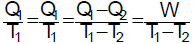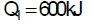The entropy change during heat addition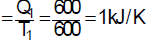QUESTION: 23

Isentropic flow is

Solution:
QUESTION: 24

A cyclic process ABCD shown in the V-T diagram performed with a constant mass of an ideal gas. The process of p-V diagram will be as shown in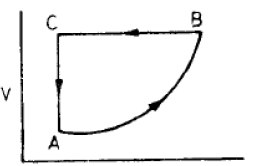Solution:

AB constant pressure heat addition.

QUESTION: 25

Assertion (A): Second law of thermodynamics is called the law of degradation of energy.

Reason (R): Energy does not degrade each time it flows through a finite temperature difference.

Solution:

A is true but R is false.

QUESTION: 26

Consider the following statements:

1. Slow heating of water from an electric heater.

2. Isentropic expansion of air.

3. Evaporation of a liquid from a heat source at the evaporation temperature.

4. Constant pressure heating of a gas by a constant temperature source.

Which of these processes is/are reversible?

Solution:

All spontaneous processes are irreversible. Statement-1 and statement-4 heat is transferred with a finite temperature difference they are irreversible.

QUESTION: 27

An inventor states that his new engine rejects to the sink 40% of heat absorbed from the source while the source and sink temperatures are 327ºC and 27ºC respectively. His engine is therefore equivalent to

Solution:

We know Carnot efficiency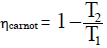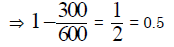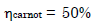But inventor claims 60% efficiency (means 40% heat rejection). It is then impossible.

QUESTION: 28

An inventor states that his new conceptual engine, while operating between temperature limits of 377oC and 27oC, will reject 50% of heat absorbed from the source. What type of cycle will this engine have?

Solution:
QUESTION: 29

An engine operates between temperature limits of 900 K and T2 and another between T2 and 400 K. For both to be equally efficient, the value of T2 will be

Solution: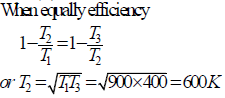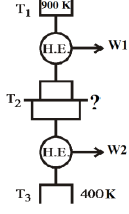QUESTION: 30

A heat engine is supplied with 250 KJ/s of heat at a constant fixed temperature of 227°C. The heat is rejected at 27°C. The cycle is reversible, if the amount of heat rejected is:

Solution: